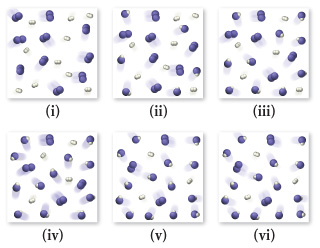# Problem: H2 and I2 are combined in a flask and allowed to react according to the following reaction: H2(g) + I2(g) ⇌ 2 HI(g)Examine the figures (sequential in time) and answer the following questions.Which figure represents the point at which equilibrium is reached?

###### FREE Expert Solution

Equilibrium → When the concentrations of reactants and products have become constant.

The reaction: H2(g) + I2(g) ⇌ 2 HI(g)

92% (316 ratings)###### Problem Details

H2 and I2 are combined in a flask and allowed to react according to the following reaction: H2(g) + I2(g) ⇌ 2 HI(g)
Examine the figures (sequential in time) and answer the following questions.Which figure represents the point at which equilibrium is reached?

Frequently Asked Questions

What scientific concept do you need to know in order to solve this problem?

Our tutors have indicated that to solve this problem you will need to apply the Chemical Equilibrium concept. You can view video lessons to learn Chemical Equilibrium. Or if you need more Chemical Equilibrium practice, you can also practice Chemical Equilibrium practice problems.

What professor is this problem relevant for?

Based on our data, we think this problem is relevant for Professor Galindo-Murillo's class at UTAH.